## More Base-8 Math Synchronicities (The Matrix, The Numbers 2117 and 3221)

This will be a continuation of the things I am being guided to in regards to the conversion of numbers from base-10 to a base-8 counting system using the numbers ‘they’ have been giving me over the years.

The first is the number that manifests when entering my birth time 11:03 into this converter: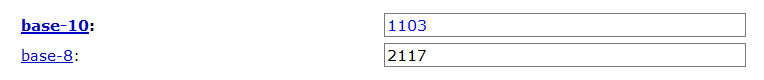After analyzing this number 2117 I found some interesting things. When we plug the number 2117 into the converter we see a 410 in the resulting number: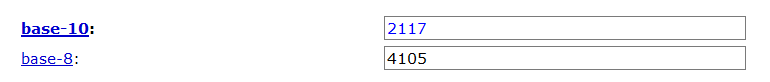And the digits of 2117 can be seen as a jumbled version of Euler’s Number which is 2.71 which is an important mathematical formula dealing with compound interest and growth and appears as ‘e’ on scientific calculators.

This number 2.71 is connected to 410 quite well. If we multiply 410 x 2.71 we get 1111.1: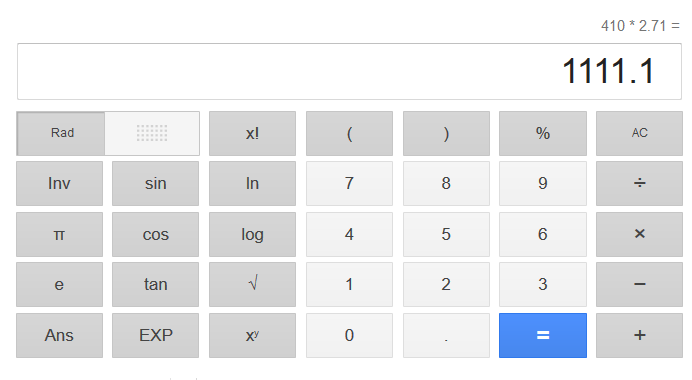If we look up the meaning of the number 2117 on the Angel’s Numbers website we see it was posted on July 21st, which if seen in number form and the day/month format would be the same digits 21/7: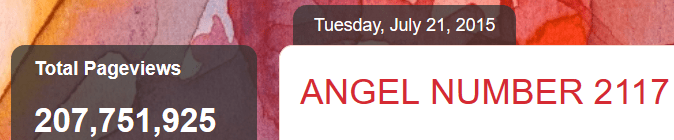And we see a 925 in the view count and we know 925 is the gematria value of ‘Jesus Christ’: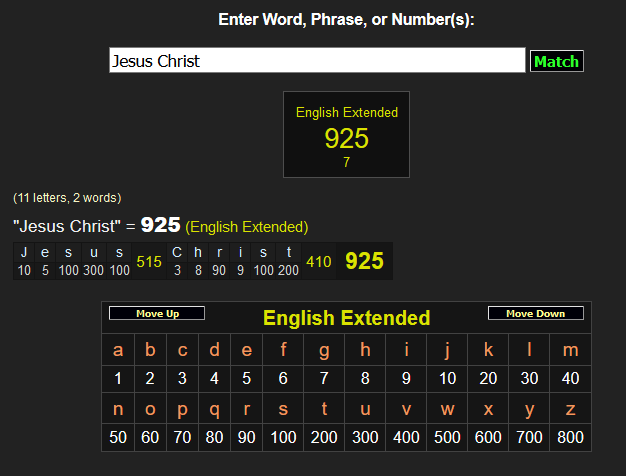And the 2117th prime number is 18481 which is 184 mirrored into itself. This combination of numbers has been appearing more lately and I am sure that will be my next set of numbers to analyze: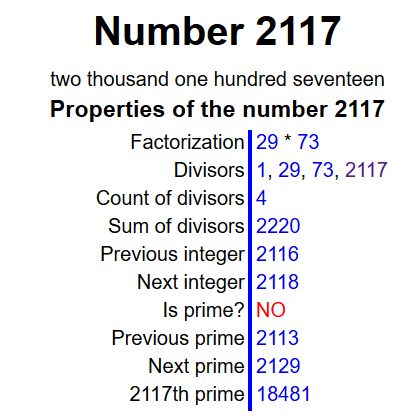For instance, the time I archived a previous post was at 1:48, in addition to this number appearing elsewhere while completing these works: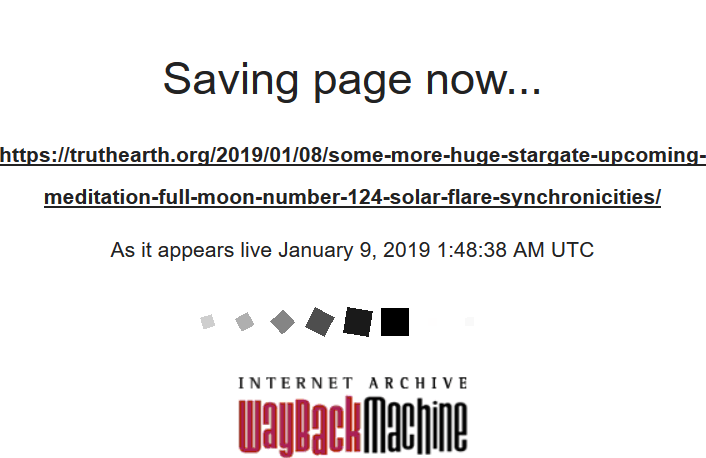The next number of interest resulted when I plugged in the number 1681 into the converter. 1681 is the root number of the result of 410 x 410 = 168100 and is a number that appears frequently:The result is the number 3221. There are a few interesting pieces of information we can pull from this number.

If we look up the meaning of the number 3221 on the Angel’s Numbers website we see a 946 in the view count and 946 as the posting time: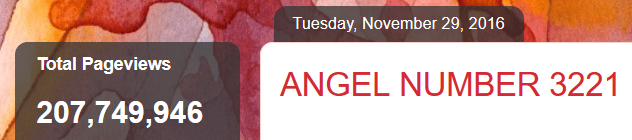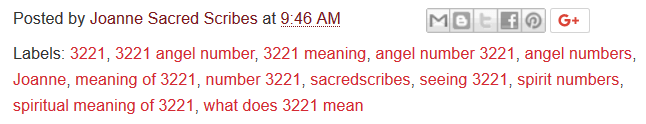The number 946 appears occasionally throughout these reports including on an electrical box near my old apartment: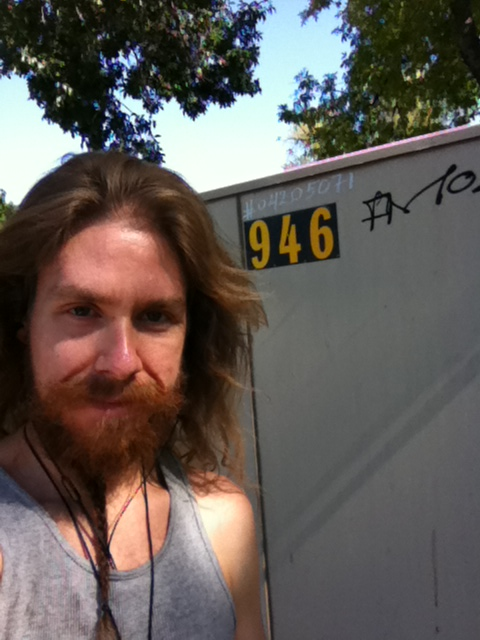Another thing to note is that the number 3221 is the number 1223 backwards and if seen as a date this would be 12/23 or December 23rd and this was the date I had the QHHT session where I was shown to be playing the role of Jesus: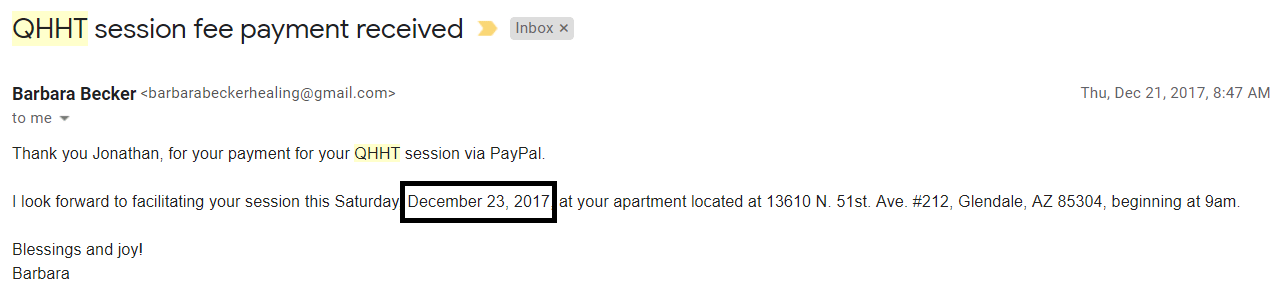The gematria value of ‘God Will Never Leave You’ is 3221: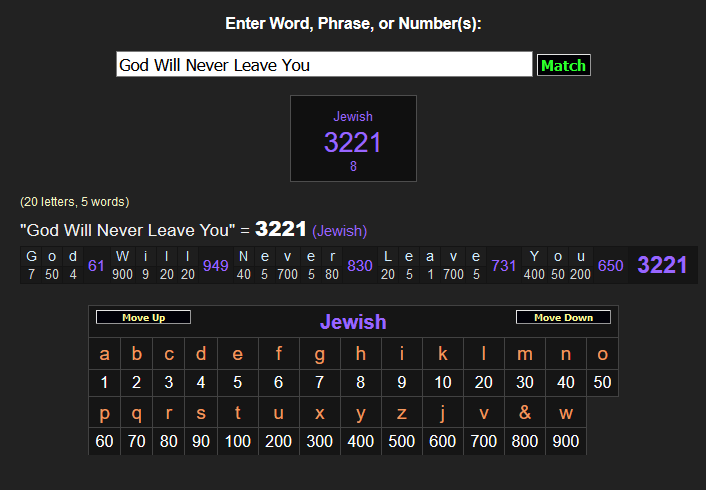If we multiply the digits of this number 3221 together (32 x 21) we get 672:These digits can be jumbled to be 726 and this is a number that has appeared recently in pretty big ways:

726 takes position 288 in Pi:And we know 288 in Pi takes position 33, the amount of years Jesus allegedly lived: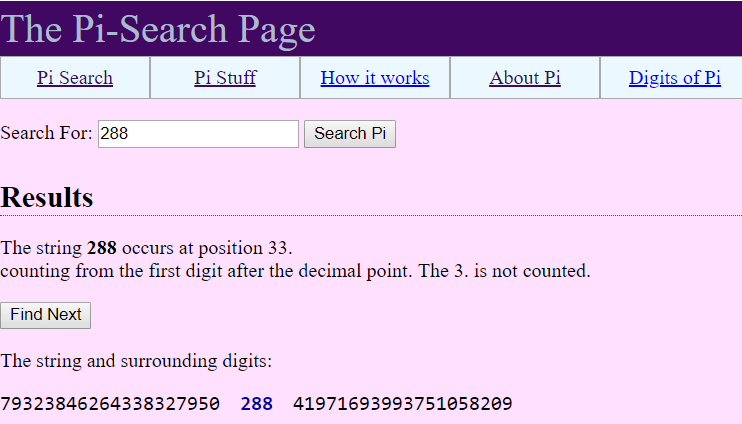And 726 is the gematria value of ‘The Messiah’ in Greek (ο Μεσσιας):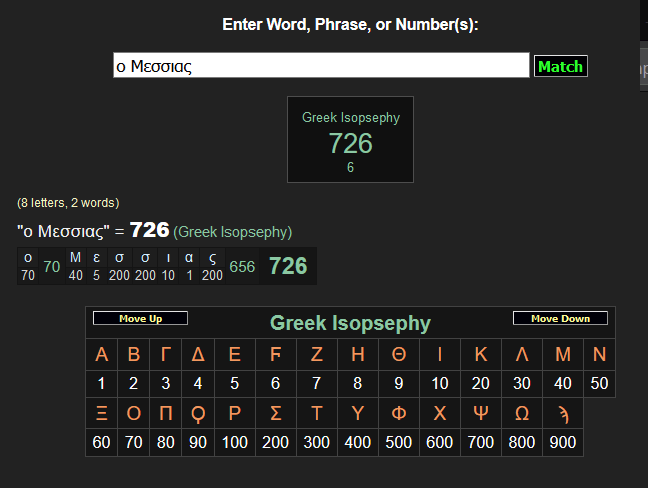If we divide the number 726 in half we get 363 which is the Hebrew gematria value of ‘The Messiah’ (המשיח):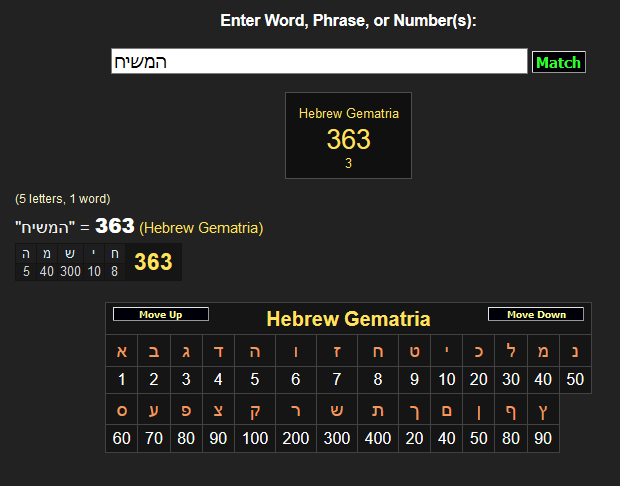If we subtract 1223 from 3221 we get 1998:And 1998 was part of a date/time stamp given in the beginning of the first Matrix movie. Additionally, if we look up the amount of weeks between April 10th, 1990 and the date provided in that beginning scene, which is February 19th, 1998, we see it is 410 weeks:I wasn’t going to add this part because I only like to include things that are right on the money and not things that get ‘close’ to the values I find here AKA ‘almost synchronicities’ but in this case it was so close I think I should.

If we subtract 2117 from 3221 we get 1104, which is one digit away from my birth time of 11:03 (another combination of the digits 1104, 4110 can be found in another recently article, perhaps that was the synchronicity):The gematria value of the phrase ‘The Window of Opportunity’ is both 331 and 3221: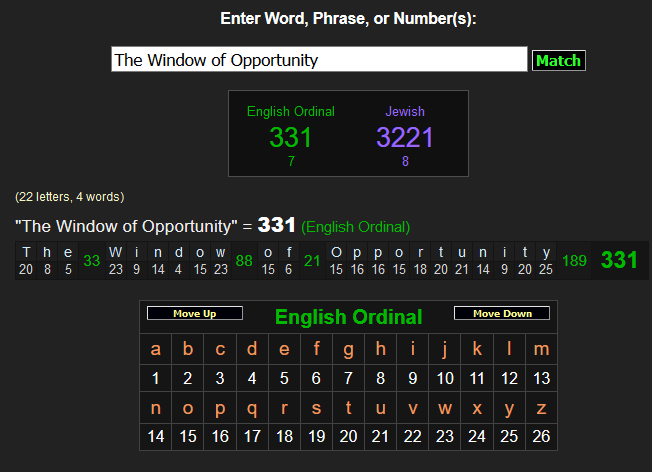And the time the meaning of the number 2117 was posted was at 3:31: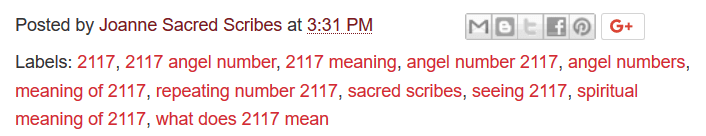And 3/31 (March 31st) was the date that the first Matrix movie premiered: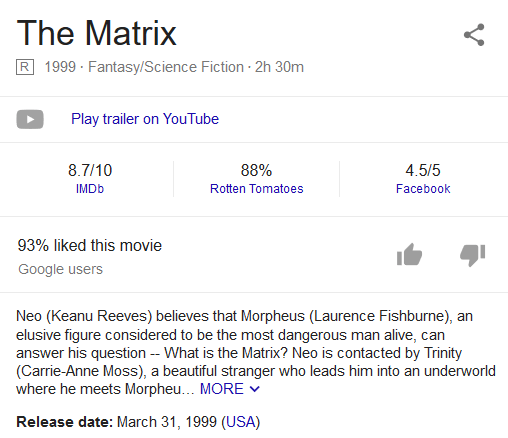3221 is the 456th prime number: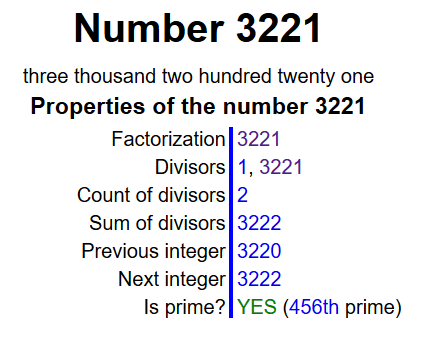And the gematria value of ‘King Messiah came’ (מלך המשיח בא) is 456: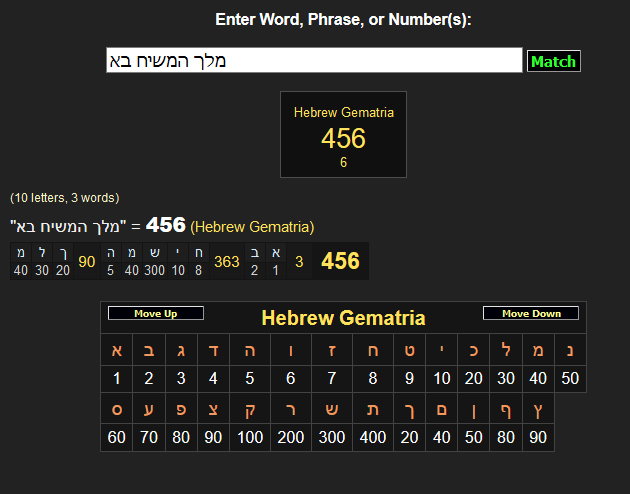If we plug in the number 456 into the converter we get 710, which, if seen as a date in day/month format would be October 7th which was the date I was shown the bible verse John 4:4 – 4:10 in a dream where the verse 4:10 talks about God sending Jesus to Earth: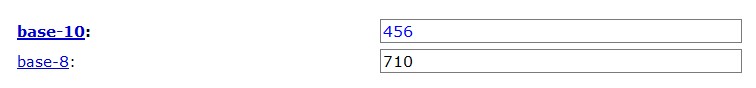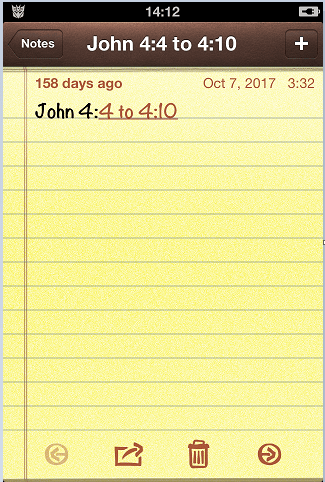And this particular post number ends in 682 which are the digits 628 in a different order and we know 628 is the result of 314 + 314 (the numbers of this post number are also all even numbers, so here we see this interesting phenomenon happening again):And I would also add that today is 1/9/19. This number 19 has a special place throughout these works and I just did a post about this number recently. It is strongly connected to information and verses of the Qur’an:

https://truthearth.org/2018/12/29/cycles-of-time-and-the-magic-number-19/

This is everything for now, much love all!

PS After being guided to plug in the above sentence I use to end these posts I found it had a gematria value of 432 (sacred geometric number)and contained 33 letters: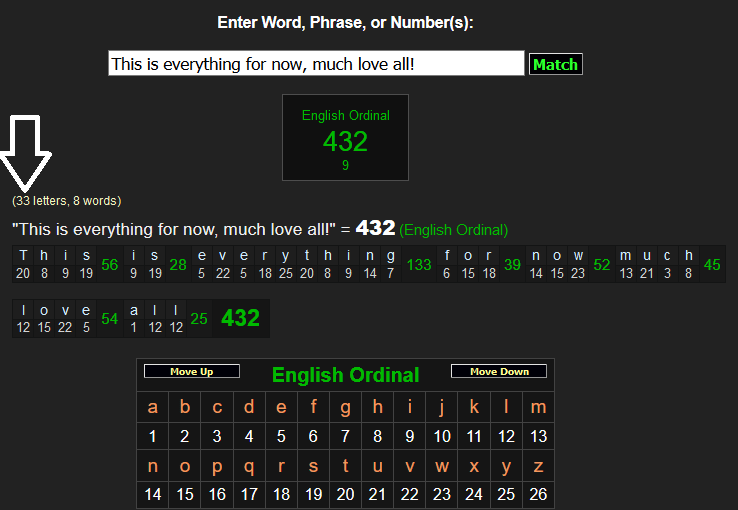This entry was posted in Uncategorized. Bookmark the permalink.